# Continuous functor

A synonym of the concept "functor commuting with limits" . Let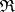and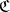be categories with limits. A one-place covariant functoris called continuous if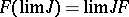for any diagram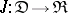with an arbitrary small diagram scheme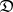. In more detail, the equality above means the following: If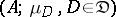is the limit of the diagram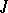and,, are morphisms occurring in the definition of limit, then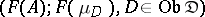is the limit of the diagram.
A functor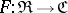is continuous if and only if it commutes with products of arbitrary families of objects and kernels of pairs of morphisms. Every basic functorfromto the category of sets is continuous.